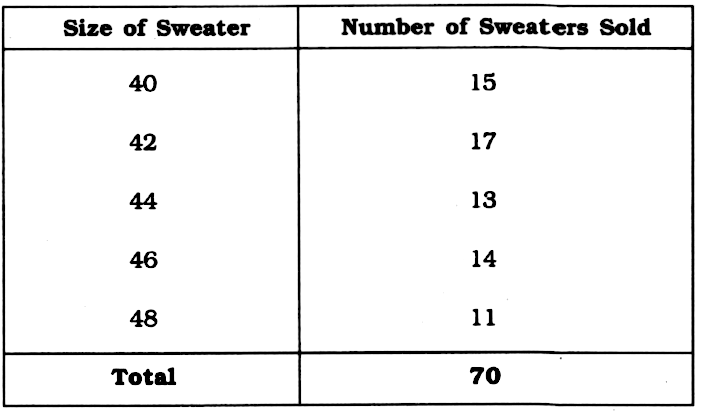Home/Class 7/Maths/

QuestionMathsClass 7

State whether the statement is True or False.In the above table, $$17$$ is the median for above data.___

×
4.64.6Solution

The sizes of sweater mentioned are $$40,\;42,\;44,\;46,\;48$$.
Thus, the median has to be a value among these given sizes.
Hence, $$17$$ can never be the median of mentioned data.